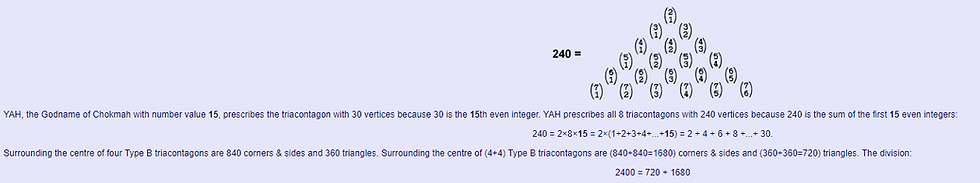top of page
Search

# tree of life superstring theory part 146

Updated: Nov 29, 2020Type A triacontagon The number of geometrical elements in a Type A/1st-order n-gon* = 4n + 1. This is because each of the n sectors adds one corner, a side of the polygon, an internal side & a triangle to its centre. A Type A triacontagon (n = 30) has 31 corners of its 30 sectors, where 31 is the number value of EL, the Godname of Chesed, and 121 geometrical elements, where 121 = 112 and 11 is the tenth integer after 1. 120 geometrical elements surround its centre, where

120 = 3 + 5 + 7 + 9 + 11 + 13 + 15 + 17 + 19 + 21

is the sum of the first ten odd integers after 1. This shows how the Decad determines the geometry of the Petrie polygon of the 421 polytope. It has 30 corners, whereType B triacontagon The number of geometrical elements in a Type B/2nd-order n-gon = 10n + 1. A Type B triacontagon has 301 geometrical elements. The number of geometrical elements in 8 concentric triacontagons = 1 + 8×300 = 2401 = 492. EL CHAI, the Godname of Yesod with number value 49, prescribes the geometrical composition of the 8 triacontagons when they are Type B. 2400 geometrical elements surround their shared centre, of which 240 are vertices and 2160 (=216×10) are corners, sides & triangles, where 216 is the number of Geburah. The number of yods surrounding the centre of the 8 triacontagons = 8×30×15 = 3600 = 36×10×10, where 36 is the number value of ELOHA, the Godname of Geburah. The number value 21 of EHYEH, the Godname of Kether, determines the number of vertices of the 8 triacontagons because 240 is the sum of the first 21 binary coefficients other than 1:is characteristic of holistic systems. For example, it manifests in the disdyakis triacontahedron as the 1680 geometrical elements that surround its axis when its faces are 0th-order triangles and as the 720 extra geometrical elements added when its faces are 1st-order triangles (see here). It is yet more evidence that the 421 polytope, whose 240 vertices project onto the corners of 8 triacontagons, constitute a holistic system. This implies, of course, that E8×E8 heterotic superstrings exist. The facts that:

• the interior angle of a triacontagon is 168° and the base angle of an isosceles sector is 84°;

• the 8 Type B triacontagons are composed of 1680 corners & sides;

• each set of 4 triacontagons comprises 840 corners & sides;

constitute persuasive evidence supporting this implication. This is because C.W. Leadbeater counted 1680 turns in each helical whorl of the UPA, its outer and inner halves having 840 turns in 2½ revolutions of a whorl around its axis, 168 turns in a half-revolution and 84 turns in a quarter-revolution. It is highly implausible that this similar set of numbers could appear by chance in the context of a paranormal account of a remote-viewed subatomic particle! Instead, it demonstrates that the 8 triacontagons, whose corners are the projections of the vertices of the 421 polytope, constitute sacred geometry that embodies both the unified forces between superstrings (namely, the 240 gauge fields associated with the 240 roots of E8) and their structure in 4-d space-time, as described with micro-psi by Besant & Leadbeater. The author is not claiming that just this property alone is the explanation for the 1680 turns in each helical whorl of the UPA. Obviously, it is only an aspect of the complete account. What he is asserting is that this number and others related to it, like 84 and 336, with a paranormal provenance do not appear by accident in the geometry of the polytope that represents the symmetry group E8 involved in E8×E8 heterotic superstring theory. That is just too improbable. On the other hand, its appearance is, precisely, what we could expect if the UPA is, indeed, a certain state of this type of superstring!

As shown in the first diagram, each sector of a Type B polygon adds 7 geometrical elements to the 3 corners & sides lining each sector of the polygon when it is Type A. This 3:7 division of the 10 geometrical elements per sector of a Type B polygon reflects the distinction in the tetractys between the 3 yods at its corners and its 7 hexagonal yods, this representing in the Tree of Life the difference between the Supernal Triad and the 7 Sephiroth of Construction. (7×240=1680) geometrical elements are added in the 8 Type B triacontagons to (3×240=720) geometrical elements in the 8 triacontagons when they are Type A. The division:

240 = 72 + 168

of the 240 roots of E8 into the 72 roots of its exceptional subgroup E6 and 168 remaining roots is an example of this. It demonstrates the deep parallels between sacred geometry and group theory. It needs to be pointed out that the superstring structural parameter 1680 appears in this calculation NOT because the Petrie polygon of the 421 polytope is the triacontagon but because E8 has 240 roots. If its Petrie polygon had been some other regular N-gon, its Coxeter projection would generate n of them, where nN = 240, so that the the number of corners & sides in the n N-gons would have been n×N×7 = 1680. So this parameter depends only on the total number of sectors of all the Petrie polygons, not on what these polygons are. In other words, it depends only on the number (240) of vertices of the 421 polytope. A Type C n-gon has 28n corners, sides & triangles surrounding its centre, so that a single triacontagon has (28×30=840) geometrical elements and all 8 triacontagons have 3360 elements. Both of these are structural parameters of the UPA. What are the chances of that occuring if the UPA had been simply an hallucination?!